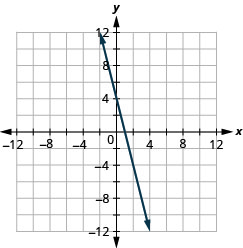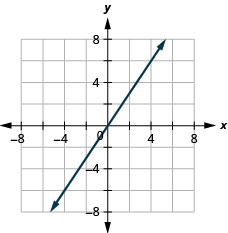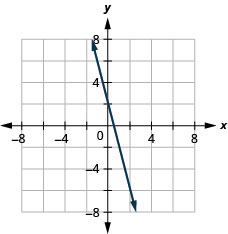# 3.1E: Exercises

•• OpenStax
• OpenStax
$$\newcommand{\vecs}{\overset { \rightharpoonup} {\mathbf{#1}} }$$ $$\newcommand{\vecd}{\overset{-\!-\!\rightharpoonup}{\vphantom{a}\smash {#1}}}$$$$\newcommand{\id}{\mathrm{id}}$$ $$\newcommand{\Span}{\mathrm{span}}$$ $$\newcommand{\kernel}{\mathrm{null}\,}$$ $$\newcommand{\range}{\mathrm{range}\,}$$ $$\newcommand{\RealPart}{\mathrm{Re}}$$ $$\newcommand{\ImaginaryPart}{\mathrm{Im}}$$ $$\newcommand{\Argument}{\mathrm{Arg}}$$ $$\newcommand{\norm}{\| #1 \|}$$ $$\newcommand{\inner}{\langle #1, #2 \rangle}$$ $$\newcommand{\Span}{\mathrm{span}}$$ $$\newcommand{\id}{\mathrm{id}}$$ $$\newcommand{\Span}{\mathrm{span}}$$ $$\newcommand{\kernel}{\mathrm{null}\,}$$ $$\newcommand{\range}{\mathrm{range}\,}$$ $$\newcommand{\RealPart}{\mathrm{Re}}$$ $$\newcommand{\ImaginaryPart}{\mathrm{Im}}$$ $$\newcommand{\Argument}{\mathrm{Arg}}$$ $$\newcommand{\norm}{\| #1 \|}$$ $$\newcommand{\inner}{\langle #1, #2 \rangle}$$ $$\newcommand{\Span}{\mathrm{span}}$$$$\newcommand{\AA}{\unicode[.8,0]{x212B}}$$

## Practice Makes Perfect

Plot Points in a Rectangular Coordinate System

In the following exercises, plot each point in a rectangular coordinate system and identify the quadrant in which the point is located.

1. a. $$(−4,2)$$ b. $$(−1,−2)$$ c. $$(3,−5)$$ d. $$(−3,0)$$
e. $$(53,2)$$2. a. $$(−2,−3)$$ b. $$(3,−3)$$ c. $$(−4,1)$$ d. $$(4,−1)$$
e. $$(32,1)$$

3. a. $$(3,−1)$$ b. $$(−3,1)$$ c. $$(−2,0)$$ d. $$(−4,−3)$$
e. $$(1,145)$$4. a. $$(−1,1)$$ b. $$(−2,−1)$$ c. $$(2,0)$$ d. $$(1,−4)$$
e. $$(3,72)$$

In the following exercises, for each ordered pair, decide

a. is the ordered pair a solution to the equation? b. is the point on the line?

5. $$y=x+2$$;

A: $$(0,2)$$; B: $$(1,2)$$; C: $$(−1,1)$$; D: $$(−3,−1)$$.a. A: yes, B: no, C: yes, D: yes b. A: yes, B: no, C: yes, D: yes

6. $$y=x−4$$;

A: $$(0,−4)$$; B: $$(3,−1)$$; C: $$(2,2)$$; D: $$(1,−5)$$.7. $$y=12x−3$$;
A: $$(0,−3)$$; B: $$(2,−2)$$; C: $$(−2,−4)$$; D: $$(4,1)$$.a. A: yes, B: yes, C: yes, D: no b. A: yes, B: yes, C: yes, D: no

8. $$y=13x+2$$;
A: $$(0,2)$$; B: $$(3,3)$$; C: $$(−3,2)$$; D: $$(−6,0)$$.Graph a Linear Equation by Plotting Points

In the following exercises, graph by plotting points.

9. $$y=x+2$$10. $$y=x−3$$

11. $$y=3x−1$$12. $$y=−2x+2$$

13. $$y=−x−3$$14. $$y=−x−2$$

15. $$y=2x$$16. $$y=−2x$$

17. $$y=12x+2$$18. $$y=13x−1$$

19. $$y=43x−5$$20. $$y=32x−3$$

21. $$y=−25x+1$$22. $$y=−45x−1$$

23. $$y=−32x+2$$24. $$y=−53x+4$$

Graph Vertical and Horizontal lines

In the following exercises, graph each equation.

25. a. $$x=4$$ b. $$y=3$$

a.b.26. a. $$x=3$$ b. $$y=1$$

27. a. $$x=−2$$ b. $$y=−5$$

a.b.28. a. $$x=−5$$ b. $$y=−2$$

In the following exercises, graph each pair of equations in the same rectangular coordinate system.

29. $$y=2x$$ and $$y=2$$30. $$y=5x$$ and $$y=5$$

31. $$y=−12x$$ and $$y=−12$$32. $$y=−13x$$ and $$y=−13$$

Find x- and y-Intercepts

In the following exercises, find the x- and y-intercepts on each graph.

33.$$(3,0),(0,3)$$

34.35.$$(5,0),(0,−5)$$

36.In the following exercises, find the intercepts for each equation.

37. $$x−y=5$$

$$x$$-int: $$(5,0)$$, $$y$$-int: $$(0,−5)$$

38. $$x−y=−4$$

39. $$3x+y=6$$

$$x$$-int: $$(2,0)$$, $$y$$-int: $$(0,6)$$

40. $$x−2y=8$$

41. $$4x−y=8$$

$$x$$-int: $$(2,0)$$, $$y$$-int: $$(0,−8)$$

42. $$5x−y=5$$

43. $$2x+5y=10$$

$$x$$-int: $$(5,0)$$, $$y$$-int: $$(0,2)$$

44. $$3x−2y=12$$

Graph a Line Using the Intercepts

In the following exercises, graph using the intercepts.

45. $$−x+4y=8$$46. $$x+2y=4$$

47. $$x+y=−3$$48. $$x−y=−4$$

49. $$4x+y=4$$50. $$3x+y=3$$

51. $$3x−y=−6$$52. $$2x−y=−8$$

53. $$2x+4y=12$$54. $$3x−2y=6$$

55. $$2x−5y=−20$$56. $$3x−4y=−12$$

57. $$y=−2x$$58. $$y=5x$$

59. $$y=x$$60. $$y=−x$$

Mixed Practice

In the following exercises, graph each equation.

61. $$y=32x$$62. $$y=−23x$$

63. $$y=−12x+3$$64. $$y=14x−2$$

65. $$4x+y=2$$66. $$5x+2y=10$$

67. $$y=−1$$68. $$x=3$$

## Writing Exercises

69. Explain how you would choose three x-values to make a table to graph the line $$y=15x−2$$.

70. What is the difference between the equations of a vertical and a horizontal line?

71. Do you prefer to use the method of plotting points or the method using the intercepts to graph the equation $$4x+y=−4$$? Why?

72. Do you prefer to use the method of plotting points or the method using the intercepts to graph the equation $$y=23x−2$$? Why?

## Self Check

a. After completing the exercises, use this checklist to evaluate your mastery of the objectives of this section.b. If most of your checks were:

Confidently. Congratulations! You have achieved the objectives in this section. Reflect on the study skills you used so that you can continue to use them. What did you do to become confident of your ability to do these things? Be specific.

With some help. This must be addressed quickly because topics you do not master become potholes in your road to success. In math every topic builds upon previous work. It is important to make sure you have a strong foundation before you move on. Who can you ask for help? Your fellow classmates and instructor are good resources. Is there a place on campus where math tutors are available? Can your study skills be improved?

No, I don’t get it. This is a warning sign and you must address it. You should get help right away or you will quickly be overwhelmed. See your instructor as soon as you can to discuss your situation. Together you can come up with a plan to get you the help you need.

This page titled 3.1E: Exercises is shared under a CC BY license and was authored, remixed, and/or curated by OpenStax.